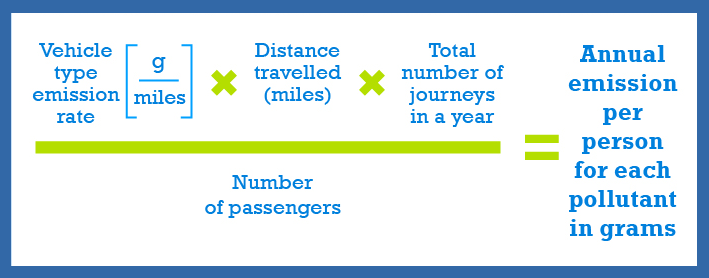# Emissions Calculator

## How we do the calculation

This calculator provides information on the annual quantity of oxides of nitrogen (NOx) and fine particulate matter (PM10) emitted to air by your journeys to and from school during a year.

To calculate how much of each pollutant is emitted to air by your school journeys we use vehicle emission factors that are based on our current understanding of emissions from vehicles in Wales.

The factors are used to calculate pollutant emission rates in grams per distance travelled for different vehicle types. The emission rate is then multiplied by the number of journeys to and from school in a year; and divided by the number of passengers in the vehicle to calculate an annual emission per person for each pollutant in grams.

The equation for the calculation is:### Assumptions made in the calculator

There are a wide number of variables used when calculating vehicle pollutant emissions so some assumptions have been made for this simplified emissions calculator as follows:

• All vehicle emission rates are calculated based on the current understanding of the Welsh vehicle fleet age mix in 2014
• School buses are assumed to be double-decker with an average of 60 passengers
• Annual emission calculations are based on a typical school year of 190 days.
• The average speed for as school journey will be 30 mph in an urban area